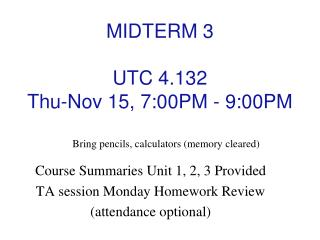DownloadDownload PresentationMIDTERM 3 UTC 4.132 Thu-Nov 15, 7:00PM - 9:00PM

# MIDTERM 3 UTC 4.132 Thu-Nov 15, 7:00PM - 9:00PM

Download Presentation## MIDTERM 3 UTC 4.132 Thu-Nov 15, 7:00PM - 9:00PM

- - - - - - - - - - - - - - - - - - - - - - - - - - - E N D - - - - - - - - - - - - - - - - - - - - - - - - - - -
##### Presentation Transcript

1. MIDTERM 3 UTC 4.132 Thu-Nov 15, 7:00PM - 9:00PM Bring pencils, calculators (memory cleared) Course Summaries Unit 1, 2, 3 Provided TA session Monday Homework Review (attendance optional)

2. Chapter 24 Classical Theory of Electromagnetic Radiation

3. Maxwell’s Equations Gauss’s law for electricity Gauss’s law for magnetism Complete Faraday’s law Ampere’s law (Incomplete Ampere-Maxwell law)

4. Ampere’s Law Current pierces surface No current inside

5. Maxwell’s Approach I ‘equivalent’ current Time varying magnetic field leads to curly electric field. Time varying electric field leads to curly magnetic field? combine with current in Ampere’s law

6. The Ampere-Maxwell Law Works!

7. Maxwell’s Equations Four equations (integral form) : Gauss’s law Gauss’s law for magnetism Faraday’s law Ampere-Maxwell law + Lorentz force

8. Fields Without Charges Time varying magnetic field makes electric field Time varying electric field makes magnetic field Do we need any charges around to sustain the fields? Is it possible to create such a time varying field configuration which is consistent with Maxwell’s equation? Solution plan: • Propose particular configuration • Check if it is consistent with Maxwell’s eqs • Show the way to produce such field • Identify the effects such field will have on matter • Analyze phenomena involving such fields

9. A Simple Configuration of Traveling Fields Key idea: Fields travel in space at certain speed Disturbance moving in space – a wave? 1. Simplest case: a pulse (moving slab)

10. A Pulse and Gauss’s Laws Pulse is consistent with Gauss’s law Pulse is consistent with Gauss’s law for magnetism

11. A Pulse and Faraday’s Law Area does not move emf Since pulse is ‘moving’, B depends on time and thus causes E E=Bv Is direction right?

12. A Pulse: Speed of Propagation E=Bv E=cB Based on Maxwell’s equations, pulse must propagate at speed of light

13. Question At this instant, the magnetic flux Fmag through the entire rectangle is: • B; B) Bx; C) Bwh; D) Bxh; E) Bvh

14. Question In a time Dt, what is DFmag? A) 0; B) BvDt; C) BhvDt; D) Bxh; E) B(x+vDt)h

15. Question emf = DFmag/Dt = ? A) 0; B) Bvh; C) Bv; D) Bxh; E) B(x+v)h

16. Question What is around the full rectangular path? • Eh; B) Ew+Eh; C) 2Ew+2Eh; D) Eh+2Ex+2EvDt; E)2EvDt

17. Question What is E? A) Bvh; B) Bv; C) Bvh/(2h+2x); D) B; E) Bvh/x

18. Exercise If the magnetic field in a particular pulse has a magnitude of 1x10-5 tesla (comparable to the Earth’s magnetic field), what is the magnitude of the associated electric field? Force on charge q moving with velocity v perpendicular to B:

19. Direction of Propagation Direction of speed is given by vector product

20. Accelerated Charges Electromagnetic pulse can propagate in space How can we initiate such a pulse? Short pulse of transverse electric field

21. Accelerated Charges E v B • Transverse pulse propagates at speed of light • Since E(t) there must be B • Direction of v is given by:

22. Magnitude of the Transverse Electric Field Field ~ -qa 1.The direction of the field is opposite toqa We can qualitatively predict the direction. What is the magnitude? Magnitude can be derived from Gauss’s law 2. The electric field falls off at a rate 1/r

23. Field of an accelerated charge Accelerates for t, then coasts for T at v=at to reach B. 1 r>cT; outer shell cT 4 inner shell of acceleration zone A > since B is closer, but = since areas compensate B 3 No charge vT ct 2

24. Field of an accelerated charge 1 cT 4 A B 3 c vT ct 2